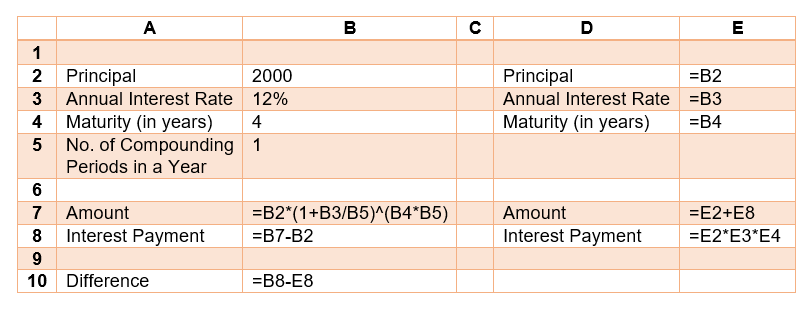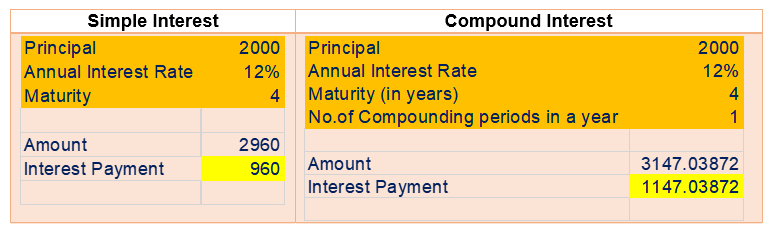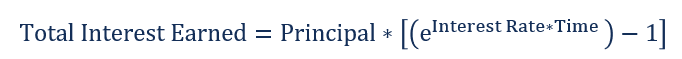# Simple Interest vs Compound Interest

Learn about the different types of interest

## What is Simple Interest vs Compound Interest?

In this article, we will discuss simple interest vs compound interest and illustrate the major differences that can arise between them. Interest payments can be thought of as the price of borrowing funds in the market. It is paid by the borrower to the lender with the payment made at the end of the loan period. Interest payments are usually calculated as a proportion of the principal that the borrower borrowed from the lender.### Summary:

• Interest payments can be thought of as the price of borrowing funds in the market. It is paid by the borrower to the lender with the payment made at the end of the loan period.
• Simple interest calculates the total interest payment using a fixed principal amount. The interest that is accrued over time is not added to the principal amount.
• Compound interest calculates the total interest payment using a variable principal amount. The interest that is accrued over time is added to the principal amount.

### What is Simple Interest?

Simple interest calculates the total interest payment using a fixed principal amount. The interest that is accrued over time is not added to the principal amount. Consider the following example:

An investor invests \$2,000 in a 4-year term deposit paying a simple interest of 12%.

Total Interest Earned = Principal * Interest Rate * Time

= \$2,000 * 12% * 4 = \$960

Average Annual Interest Earned = Total Interest Earned / Time

= \$960 / 4 = \$240

Total Amount Repaid = Principal + Total Interest

= \$2,000 + \$960 = \$2,960

### What is Compound Interest?

Compound interest calculates the total interest payment using a variable principal amount. The interest that is accrued over time is added to the principal amount. For example, the interest for the first year is calculated as a proportion of the initial principal. The interest amount is then added to the initial principal, and the interest for the second year is calculated as a proportion of the revised principal. Consider the following example:

An investor invests \$2,000 in a 4-year term deposit paying an annual interest of 12% with interest compounded annually.Where:

• N is the number of times in a year the interest is compounded or added to the initial principal.

Total Interest Earned = \$2,000 * [(1 + 12%)4 – 1] = \$1,147.04

Average Annual Interest Earned = Total Interest Earned / Time

= \$1,147.04 / 4 = \$286.76

### Simple Interest vs. Compound Interest

The following Excel spreadsheet can be used to illustrate the large differences between simple interest and compound interest payments:### Continuous Compounding

In the example above, interest was compounded on an annual basis. However, we could’ve just as easily compounded on a semi-yearly or a quarter-yearly basis. In fact, we could’ve also compounded the interest every day or every minute or even every second.

Continuous compounding recalculates the principal on a continuous basis. Continuously compounded interest can be found using the following formula:Where:

• e is Euler’s number ≈ 2.7183

Continuing with the example above, if \$2,000 is lent out for 4 years at an annual interest rate of 12% and the interest is compounded continuously, the total interest earned is \$1,232.15. The result can be verified by setting the number of compounding periods in the Excel spreadsheet to a very large number (such as 100,000).

### More Resources

CFI is the official provider of the global Financial Modeling & Valuation Analyst (FMVA)™ certification program, designed to help anyone become a world-class financial analyst. To keep advancing your career, the additional resources below will be useful:

• Annual Percentage Rate (APR)
• Continuously Compounded Interest
• Effective Annual Interest Rate
• Interest Payable

### Financial Analyst Training

Get world-class financial training with CFI’s online certified financial analyst training program!

Gain the confidence you need to move up the ladder in a high powered corporate finance career path.

Learn financial modeling and valuation in Excel the easy way, with step-by-step training.